Uh Oh! It seems you’re using an Ad blocker!

Since we’ve struggled a lot to makes online calculations for you, we are appealing to you to grant us by disabling the Ad blocker for this domain.# Triangle Calculator

Please put 3 values containing at least one side to the following six (6) fields, and hit the 'Calculate' button to see the results. While radians are selected as an angle unit, as well as it can take different values such as pi/2, pi/4.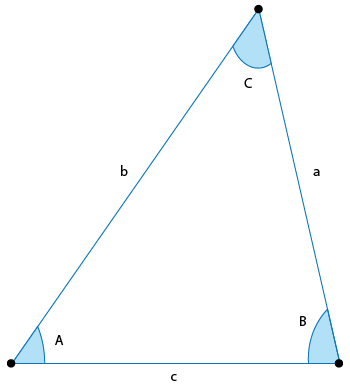a:

b:

c:

A:

B:

C:

Angle in:

Table of Content

 1 What Is A Triangle? 2 Types Of Triangles: 3 Important Facts About Triangles: 4 Formula For Triangle Terms: 5 How To Calculate Terms Associated With The Triangle? 6 What do you know about an oblique triangle? 7 What are the basic trigonometric formulas? 8 What are the six trigonometric functions?

Get the Widget!

Add this calculator to your site and lets users to perform easy calculations.

Feedback

How easy was it to use our calculator? Did you face any problem, tell us!

An online triangle calculator is exclusively programmed to determine each and every term associated with this geometrical figure. This content is arranged for you people so that you may not face any difficulty in using this free triangle solver. Also, you will get a detailed context regarding all terms to understand them better.

Simply stay focused!

## What Is A Triangle?

In geometrical analysis:

“A polygon having only three sides is considered a triangle”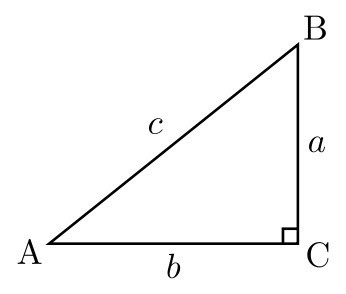Let us understand some basics first:

Vertex:

“A particular point where two sides meet with each other is called vertex”.

In a triangle, we have three sides that are connected to each other. In this way, we have three vertices A, B, and C for every triangle which are also known as ‘edges’

Angle:

“An angle is formed by joining an incident ray with the reflected ray”.

We have three triangle angles designated as ∠A, ∠B, and ∠C, respectively. These angles differ for every different types of triangles. With the help of a free angle finder calculator, you can easily find angle of triangle in a fraction of seconds.

Sides:

“The line segments joining to form a triangle are termed sides of a triangle.”

In a triangle, we have three sides as a, b, and c, respectively.

You can easily solve the triangle with all above terms known and fetching them to a free triangle side calculator.

### Types Of Triangles:

This sections falls into two categories that are properly explained below:

#### Triangle Types (Based On The Angles Of A Triangle):

Depending on the interior angles, we have three types of triangle described as follows:

Right-Angled Triangle:

“A triangle having at least one right angle is called a right-angled triangle.”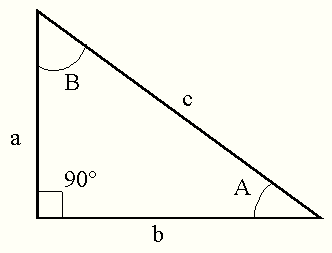Mathematically, we have:

$$m∠C = 90^\text{o}$$

A right triangle calculator can be used to analyze a right-angled triangle thoroughly.

Acute-Angled Triangle:

“A triangle whose measure of all angles is acute is called an acute-angled triangle.”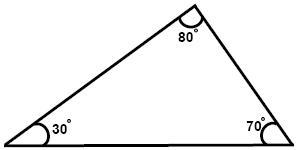Mathematically, we have:
$$m∠A < 90^\text{o}$$
$$m∠B < 90^\text{o}$$
$$m∠C < 90^\text{o}$$

Obtuse-Angled Triangle:

“A triangle having at least one angle greater than 90 degree and other two acute angles is known as an obtuse-angled triangle.”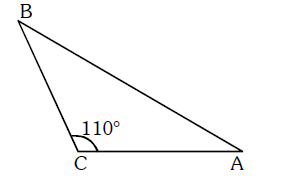Mathematically, we have:
$$m∠A >90^\text{o}$$
$$m∠B < 90^\text{o}$$
$$m∠C < 90^\text{o}$$

Subjecting to a free online triangle calculator assists you in determining all the angles for any above of the triangles.

#### Triangle Types (Based On The Sides Of A Triangle):

On the basis of triangle sides, we have following types of triangles:

Equilateral Triangle:

“If all three sides of a triangle are equal, then it is said to be an equilateral triangle”.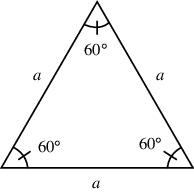Mathematically, we have:

$$m∠A = m∠B = m∠C = 60^\text{o}$$

Isosceles Triangle:

“It is a triangle whose at least two sides are equal to each other”.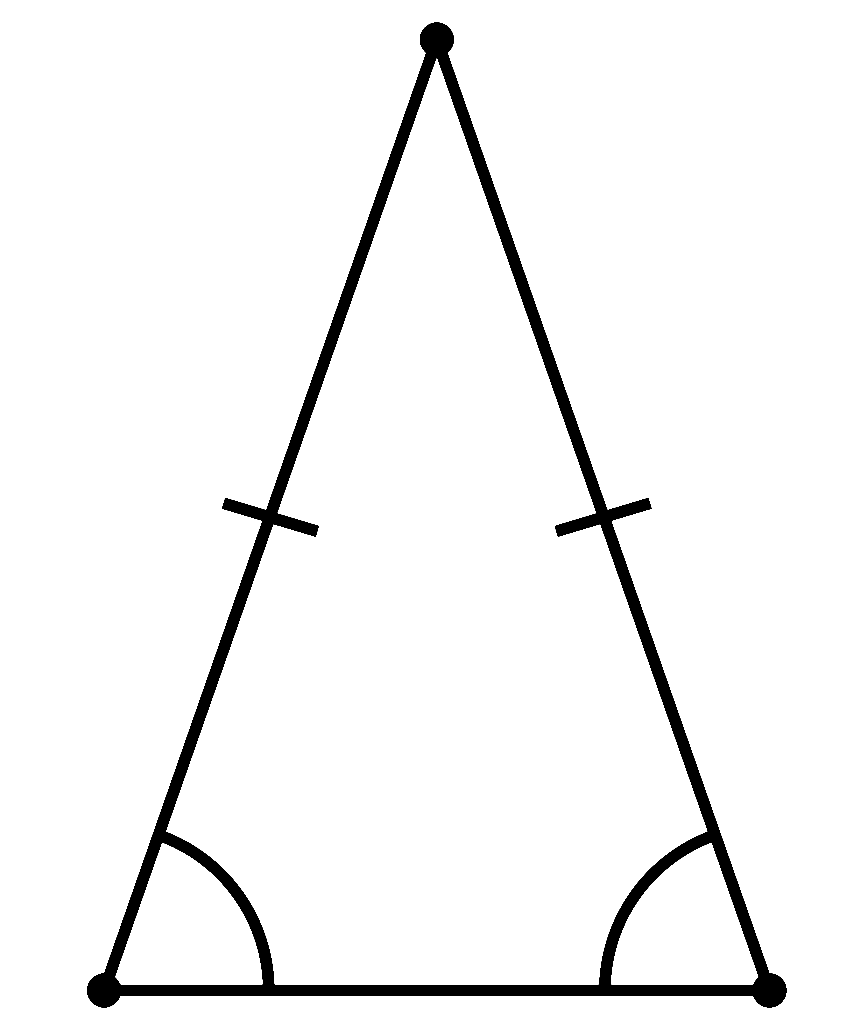The two equal sides of the isosceles triangle have a vertex angle among them. The only side that is different from two of the sides is its base.

Mathematically, we have:
$$m∠A = 38^\text{o}$$
$$m∠B = m∠C = 71^\text{o}$$

Scalene Triangle:

“It is a triangle in which all the three sides and angles are not congruent.”Mathematically, we have:
$$Side A ≠ Side B ≠ Side C$$
$$m∠A ≠ m∠B ≠ m∠C$$

Following are some key features of triangles that you need to keep in mind for sure:

Sum Of Interior Angles:

No matter what is the individual measure of all the angles of a triangle. The only fact to be considered is that their sum is always supplementary.

$$m∠A + m∠B + m∠C = 180^\text{o}$$

$$90^\text{o}$$ Angle Or Greater:

In case if the interior angles become equal to or greater than 90 degrees, then the resulting image will not be considered a triangle.

Sum Of Lengths:

If we add any two sides of the triangle, then their sum is always larger than the third side.

$$Side A + Side B > Side C$$

The Law Of Sine:

By using the law of sine, you can easily find the missing side of a triangle or missing angle of a triangle without any hurdle.

$$\frac{a}{sin\left(A\right)} = \frac{b}{sin\left(B\right)} = \frac{c}{sin\left(C\right)}$$

For a proper understanding of the law of sine, subject to the free law of sine calculator.

### Formula For Triangle Terms:

In this section, we will be discussing and mentioning the formula against each single term in a triangle that are very crucial. What you need to do is to stay focused.

#### Angles Of The Triangle:

Here we have different formulas defined for different cases.

Case 1:

When two sides a and b are given with ∠B, use the formula given below:
$$∠A = sin^{-1}(\frac{a\times sin(B)}{b})$$

Case 2:

When two sides a and b are given with ∠C, use the following formula:

## Conclusion:

A triangle geometry is a branch of mathematics in which trigonometry revolves. We can not solve any triangle without having knowledge of trigonometry. A free triangle calculator also follows the basic laws of trigonometry to solve for triangles. Professional scholars, engineers, students and even aerospace make use of triangle analysis widely to determine various terms.